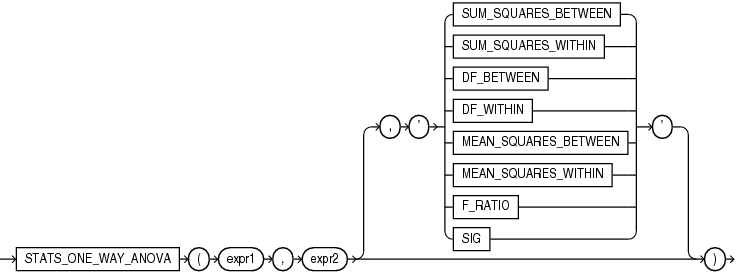## STATS_ONE_WAY_ANOVA

SyntaxDescription of the illustration stats_one_way_anova.eps

Purpose

The one-way analysis of variance function (`STATS_ONE_WAY_ANOVA`) tests differences in means (for groups or variables) for statistical significance by comparing two different estimates of variance. One estimate is based on the variances within each group or category. This is known as the mean squares within or mean square error. The other estimate is based on the variances among the means of the groups. This is known as the mean squares between. If the means of the groups are significantly different, then the mean squares between will be larger than expected and will not match the mean squares within. If the mean squares of the groups are consistent, then the two variance estimates will be about the same.

`STATS_ONE_WAY_ANOVA` takes two required arguments: `expr1` is an independent or grouping variable that divides the data into a set of groups and `expr2` is a dependent variable (a numeric expression) containing the values corresponding to each member of a group. The optional third argument lets you specify the meaning of the `NUMBER` value returned by this function, as shown in Table 7-8. For this argument, you can specify a text literal, or a bind variable or expression that evaluates to a constant character value. If you omit the third argument, then the default is `'SIG'`.

Appendix C in Oracle Database Globalization Support Guide for the collation determination rules for `STATS_ONE_WAY_ANOVA`

Table 7-8 STATS_ONE_WAY_ANOVA Return Values

Argument Return Value Meaning

`'SUM_SQUARES_BETEEN'`

Sum of squares between groups

`'SUM_SQUARES_WITHIN'`

Sum of squares within groups

`’DF_BETWEEN'`

Degree of freedom between groups

`'DF_WITHIN'`

Degree of freedom within groups

`'MEAN_SQUARES_BETWEEN'`

Mean squares between groups

`'MEAN_SQUARES_WITHIN'`

Mean squares within groups

`'F_RATIO'`

Ratio of the mean squares between to the mean squares within (MSB/MSW)

`'SIG'`

Significance

The significance of one-way analysis of variance is determined by obtaining the one-tailed significance of an f-test on the ratio of the mean squares between and the mean squares within. The f-test should use one-tailed significance, because the mean squares between can be only equal to or larger than the mean squares within. Therefore, the significance returned by `STATS_ONE_WAY_ANOVA` is the probability that the differences between the groups happened by chance—a number between 0 and 1. The smaller the number, the greater the significance of the difference between the groups. Refer to the STATS_F_TEST for information on performing an f-test.

STATS_ONE_WAY_ANOVA Example

The following example determines the significance of the differences in mean sales within an income level and differences in mean sales between income levels. The results, p_values close to zero, indicate that, for both men and women, the difference in the amount of goods sold across different income levels is significant.

```SELECT cust_gender,
STATS_ONE_WAY_ANOVA(cust_income_level, amount_sold, 'F_RATIO') f_ratio,
STATS_ONE_WAY_ANOVA(cust_income_level, amount_sold, 'SIG') p_value
FROM sh.customers c, sh.sales s
WHERE c.cust_id = s.cust_id
GROUP BY cust_gender
ORDER BY cust_gender;

C    F_RATIO    P_VALUE
- ---------- ----------
F 5.59536943 4.7840E-09
M  9.2865001 6.7139E-17```